# Introduction to ggridges

#### 2020-01-11

Ridgeline plots are partially overlapping line plots that create the impression of a mountain range. They can be quite useful for visualizing changes in distributions over time or space.

## Geoms

The ggridges package provides two main geoms, `geom_ridgeline` and `geom_density_ridges`. The former takes height values directly to draw ridgelines, and the latter first estimates data densities and then draws those using ridgelines.

### Ridgelines

The geom `geom_ridgeline` can be used to draw lines with a filled area underneath.

``````library(ggplot2)
library(ggridges)

data <- data.frame(x = 1:5, y = rep(1, 5), height = c(0, 1, 3, 4, 2))
ggplot(data, aes(x, y, height = height)) + geom_ridgeline()``````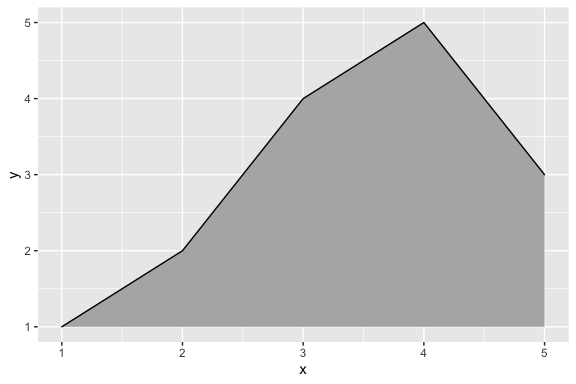Negative heights are allowed, but are cut off unless the `min_height` parameter is set negative as well.

``````library(patchwork) # for side-by-side plotting

data <- data.frame(x = 1:5, y = rep(1, 5), height = c(0, 1, -1, 3, 2))
plot_base <- ggplot(data, aes(x, y, height = height))

plot_base + geom_ridgeline() | plot_base + geom_ridgeline(min_height = -2)``````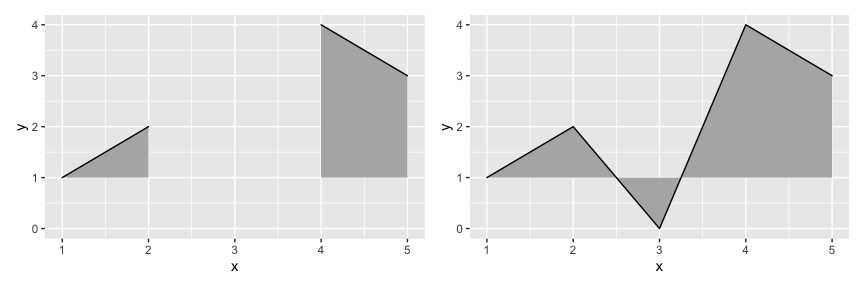Multiple ridgelines can be drawn at the same time. They will be ordered such that the ones drawn higher up are in the background. When drawing multiple ridgelines at once, the `group` aesthetic must be specified so that the geom knows which parts of the data belong to which ridgeline.

``````d <- data.frame(
x = rep(1:5, 3),
y = c(rep(0, 5), rep(1, 5), rep(2, 5)),
height = c(0, 1, 3, 4, 0, 1, 2, 3, 5, 4, 0, 5, 4, 4, 1)
)

ggplot(d, aes(x, y, height = height, group = y)) +
geom_ridgeline(fill = "lightblue")``````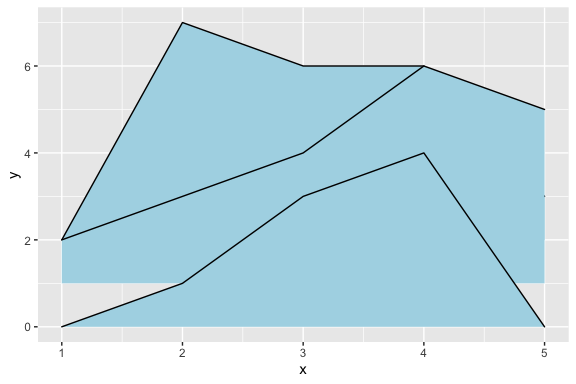It is also possible to draw ridgelines with `geom_density_ridges` if we set `stat = "identity"`. In this case, the heights are automatically scaled such that the highest ridgeline just touches the one above at `scale = 1`.

``````ggplot(d, aes(x, y, height = height, group = y)) +
geom_density_ridges(stat = "identity", scale = 1)``````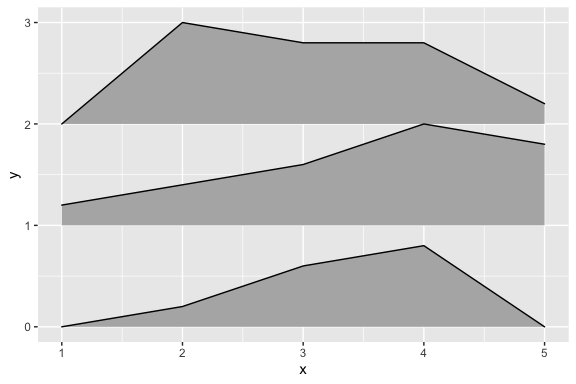### Density ridgeline plots

The geom `geom_density_ridges` calculates density estimates from the provided data and then plots those, using the ridgeline visualization. The `height` aesthetic does not need to be specified in this case.

``ggplot(iris, aes(x = Sepal.Length, y = Species)) + geom_density_ridges()``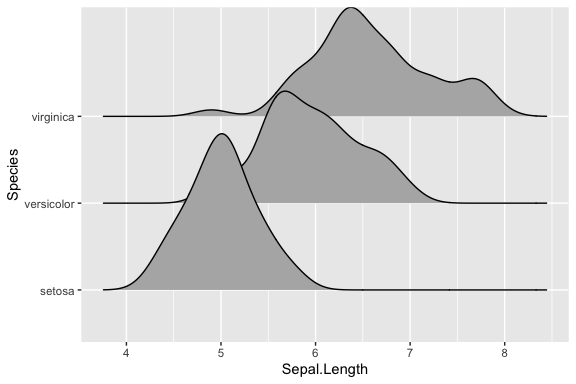There is also `geom_density_ridges2`, which is identical to `geom_density_ridges` except it uses closed polygons instead of ridgelines for drawing.

``ggplot(iris, aes(x = Sepal.Length, y = Species)) + geom_density_ridges2()``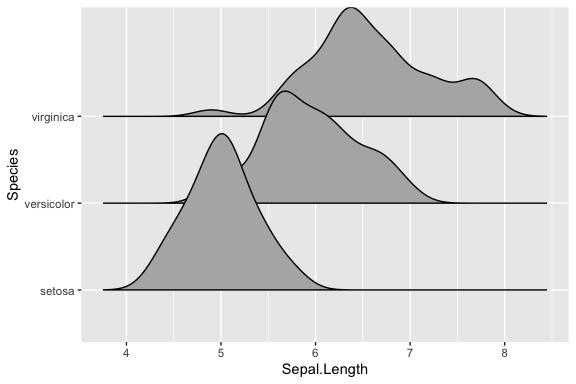The grouping aesthetic does not need to be provided if a categorical variable is mapped onto the y axis, but it does need to be provided if the variable is numerical.

``````# modified dataset that represents species as a number
iris_num <- transform(iris, Species_num = as.numeric(Species))

# does not work, causes error
# ggplot(iris_num, aes(x = Sepal.Length, y = Species)) + geom_density_ridges()

# works
ggplot(iris_num, aes(x = Sepal.Length, y = Species_num, group = Species_num)) +
geom_density_ridges()``````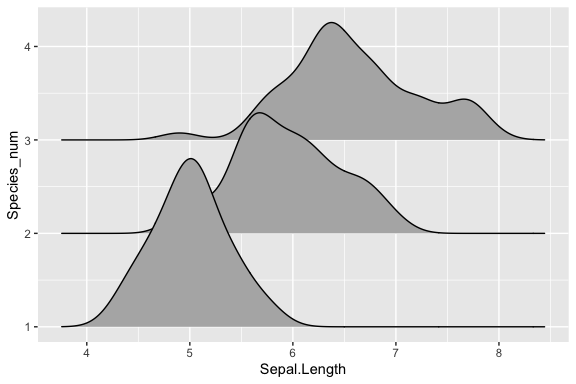Trailing tails can be cut off using the `rel_min_height` aesthetic. This aesthetic sets a percent cutoff relative to the highest point of any of the density curves. A value of 0.01 usually works well, but you may have to modify this parameter for different datasets.

``````ggplot(iris, aes(x = Sepal.Length, y = Species)) +
geom_density_ridges(rel_min_height = 0.01)``````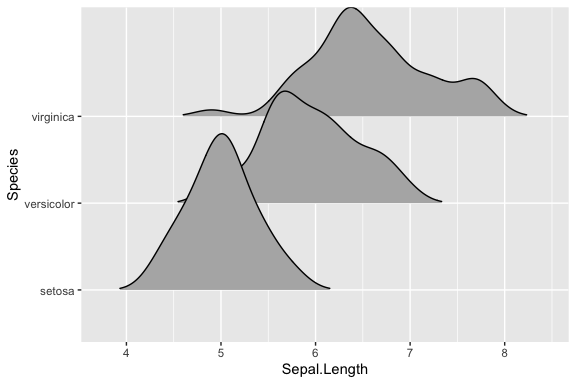The extent to which the different densities overlap can be controlled with the `scale` parameter. A setting of `scale=1` means the tallest density curve just touches the baseline of the next higher one. Smaller values create a separation between the curves, and larger values create more overlap.

``````# scale = 0.9, not quite touching
ggplot(iris, aes(x = Sepal.Length, y = Species)) + geom_density_ridges(scale = 0.9)``````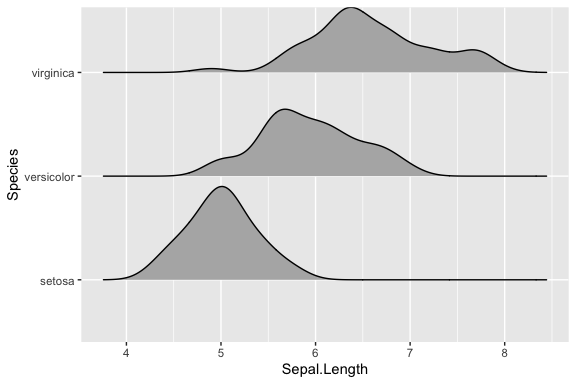``````# scale = 1, exactly touching
ggplot(iris, aes(x = Sepal.Length, y = Species)) + geom_density_ridges(scale = 1)``````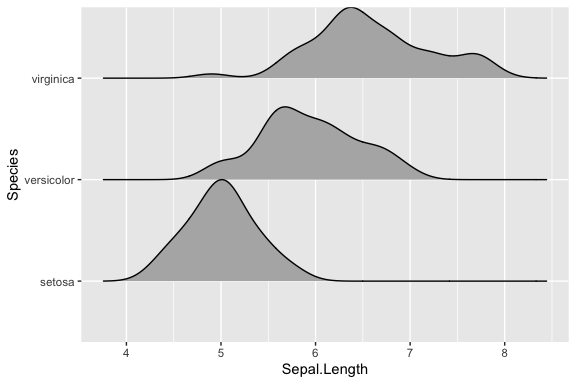``````# scale = 5, substantial overlap
ggplot(iris, aes(x = Sepal.Length, y = Species)) + geom_density_ridges(scale = 5)``````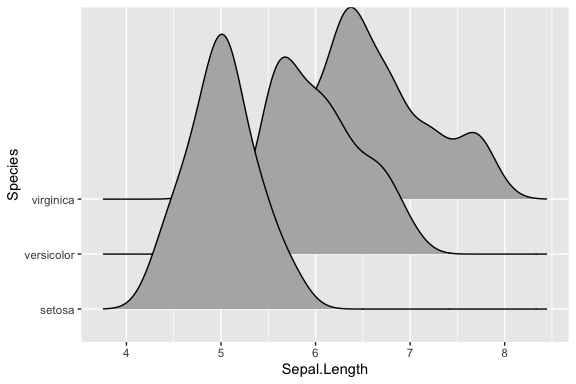The scaling is calculated separately per panel, so if we facet-wrap by species each density curve exactly touches the next higher baseline. (This can be disabled by setting `panel_scaling = FALSE`.)

``````ggplot(iris, aes(x = Sepal.Length, y = Species)) +
geom_density_ridges(scale = 1) + facet_wrap(~Species)``````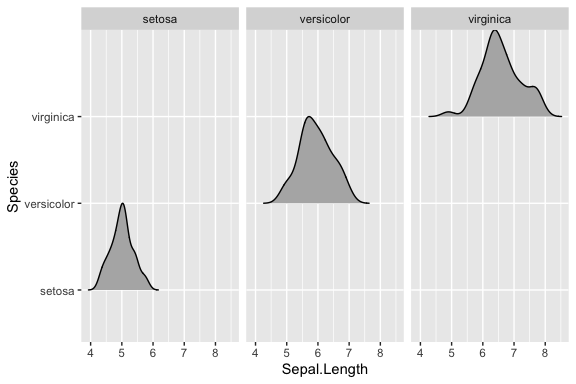### Varying fill colors along the x axis

Sometimes we would like to have the area under a ridgeline not filled with a single solid color but rather with colors that vary in some form along the x axis. This effect can be achieved with the geoms `geom_ridgeline_gradient` and `geom_density_ridges_gradient`. Both geoms work just like `geom_ridgeline` and `geom_density_ridges`, except that they allow for varying fill colors. However, they do not allow for alpha transparency in the fill. For technical reasons, we can have changing fill colors or transparency but not both.

Here is a simple example of changing fill colors with `geom_ridgeline_gradient`:

``````d <- data.frame(
x = rep(1:5, 3) + c(rep(0, 5), rep(0.3, 5), rep(0.6, 5)),
y = c(rep(0, 5), rep(1, 5), rep(3, 5)),
height = c(0, 1, 3, 4, 0, 1, 2, 3, 5, 4, 0, 5, 4, 4, 1))

ggplot(d, aes(x, y, height = height, group = y, fill = factor(x+y))) +
scale_fill_viridis_d(direction = -1, guide = "none")``````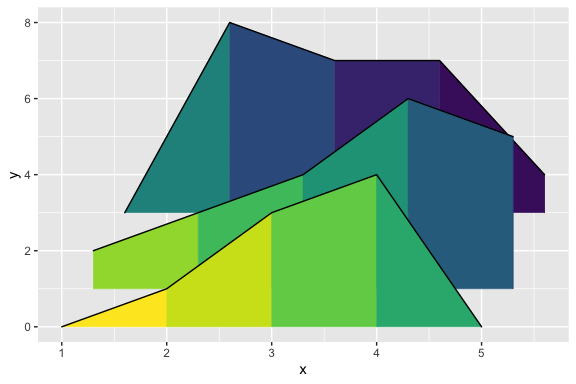And here is an example using `geom_density_ridges_gradient`. Note that we need to map the calculated x value (`stat(x)`) onto the fill aesthetic, not the original temperature variable. This is the case because `geom_density_ridges_gradient` calls `stat_density_ridges` (described in the next section) which calculates new x values as part of its density calculation.

``````ggplot(lincoln_weather, aes(x = `Mean Temperature [F]`, y = Month, fill = stat(x))) +
geom_density_ridges_gradient(scale = 3, rel_min_height = 0.01) +
scale_fill_viridis_c(name = "Temp. [F]", option = "C") +
labs(title = 'Temperatures in Lincoln NE in 2016')``````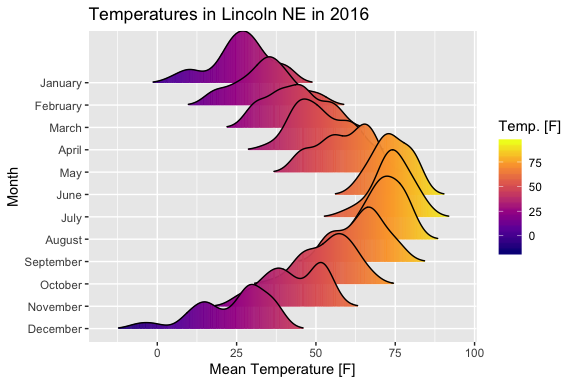## Stats

The ggridges package provides a stat `stat_density_ridges` that replaces `stat_density` in the context of ridgeline plots. In addition to setting up the proper `height` for `geom_density_ridges`, this stat has a number of additional features that may be useful.

### Quantile lines and coloring by quantiles or probabilities

By setting the option `quantile_lines = TRUE`, we can make `stat_density_ridges` calculate the position of lines indicating quantiles. By default, three lines are drawn, corresponding to the first, second, and third quartile:

``````ggplot(iris, aes(x = Sepal.Length, y = Species)) +
stat_density_ridges(quantile_lines = TRUE)``````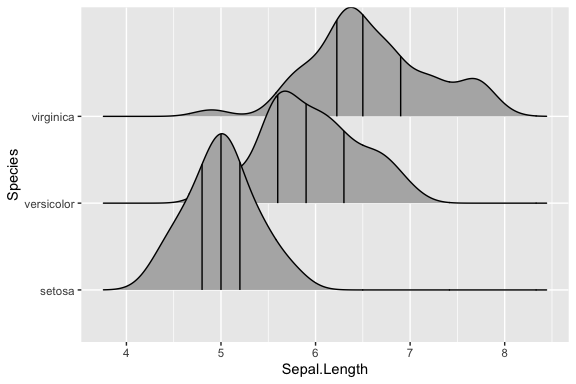We can change the number of quantiles by specifying it via the `quantiles` option. Note that `quantiles = 2` implies one line (the median) at the boundary between the two quantiles.

``````ggplot(iris, aes(x = Sepal.Length, y = Species)) +
stat_density_ridges(quantile_lines = TRUE, quantiles = 2)``````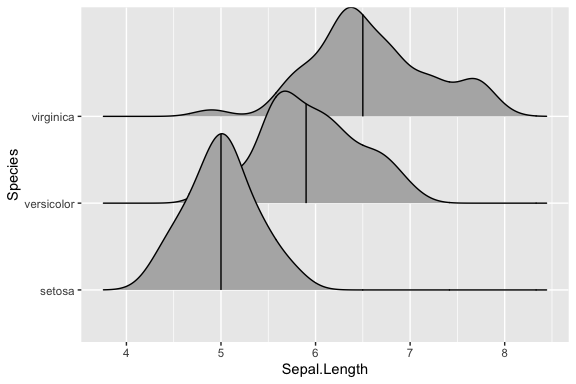We can also specify quantiles by cut points rather than number. E.g., we can indicate the 2.5% and 97.5% tails.

``````ggplot(iris, aes(x = Sepal.Length, y = Species)) +
stat_density_ridges(quantile_lines = TRUE, quantiles = c(0.025, 0.975), alpha = 0.7)``````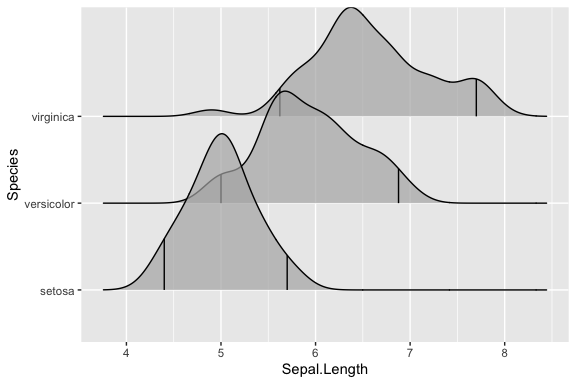Using the geom `geom_density_ridges_gradient` we can also color by quantile, via the calculated `stat(quantile)` aesthetic. Note that this aesthetic is only calculated if `calc_ecdf = TRUE`.

``````ggplot(iris, aes(x=Sepal.Length, y=Species, fill = factor(stat(quantile)))) +
stat_density_ridges(
geom = "density_ridges_gradient", calc_ecdf = TRUE,
quantiles = 4, quantile_lines = TRUE
) +
scale_fill_viridis_d(name = "Quartiles")``````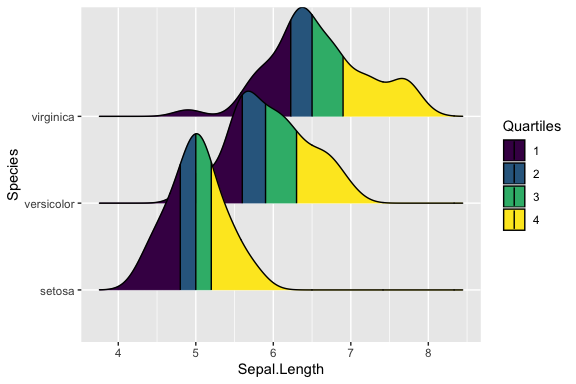We can use the same approach to highlight the tails of the distributions.

``````ggplot(iris, aes(x = Sepal.Length, y = Species, fill = factor(stat(quantile)))) +
stat_density_ridges(
calc_ecdf = TRUE,
quantiles = c(0.025, 0.975)
) +
scale_fill_manual(
name = "Probability", values = c("#FF0000A0", "#A0A0A0A0", "#0000FFA0"),
labels = c("(0, 0.025]", "(0.025, 0.975]", "(0.975, 1]")
)``````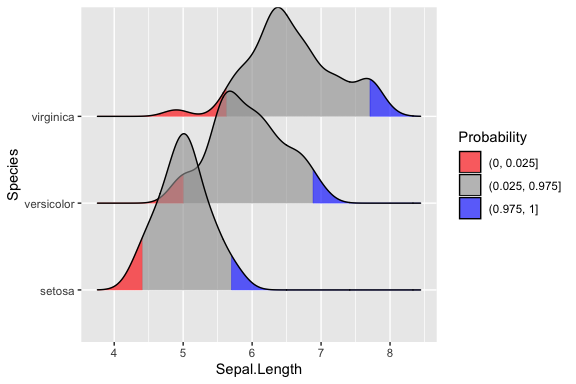Finally, when `calc_ecdf = TRUE`, we also have access to a calculated aesthetic `stat(ecdf)`, which represents the empirical cumulative density function for the distribution. This allows us to map the probabilities directly onto color.

``````ggplot(iris, aes(x = Sepal.Length, y = Species, fill = 0.5 - abs(0.5 - stat(ecdf)))) +
stat_density_ridges(geom = "density_ridges_gradient", calc_ecdf = TRUE) +
scale_fill_viridis_c(name = "Tail probability", direction = -1)``````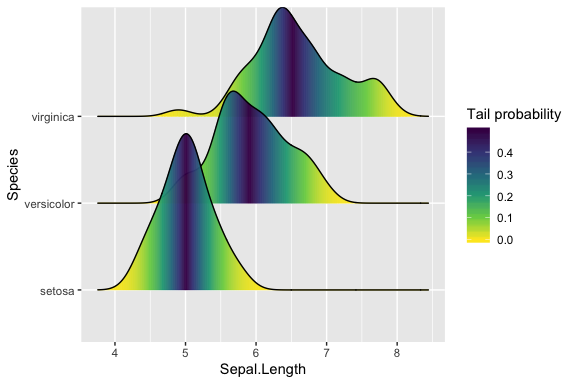### Jittering points

The stat `stat_density_ridges` also provides the option to visualize the original data points from which the distributions are generated. This can be done by setting `jittered_points = TRUE`, either in `stat_density_ridges` or in `geom_density_ridges`:

``````ggplot(iris, aes(x = Sepal.Length, y = Species)) +
geom_density_ridges(jittered_points = TRUE)``````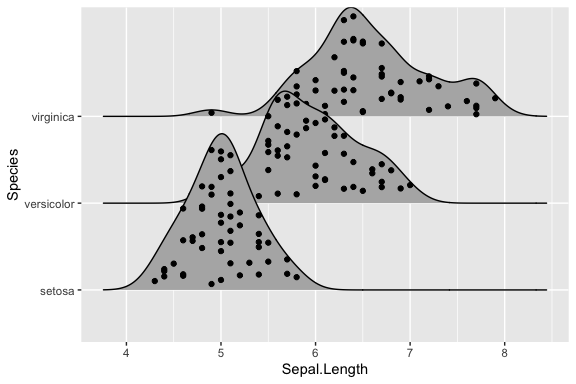Where the points are shown can be controlled with position options, e.g. “raincloud” for the raincloud effect:

``````ggplot(iris, aes(x = Sepal.Length, y = Species)) +
geom_density_ridges(
jittered_points = TRUE, position = "raincloud",
alpha = 0.7, scale = 0.9
)``````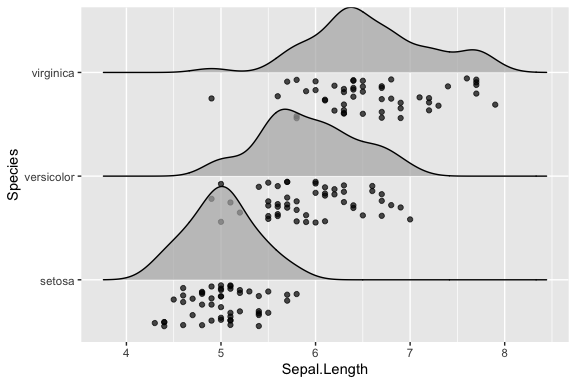We can also simulate a rug:

``````ggplot(iris, aes(x = Sepal.Length, y = Species)) +
geom_density_ridges(
jittered_points = TRUE,
position = position_points_jitter(width = 0.05, height = 0),
point_shape = '|', point_size = 3, point_alpha = 1, alpha = 0.7,
)``````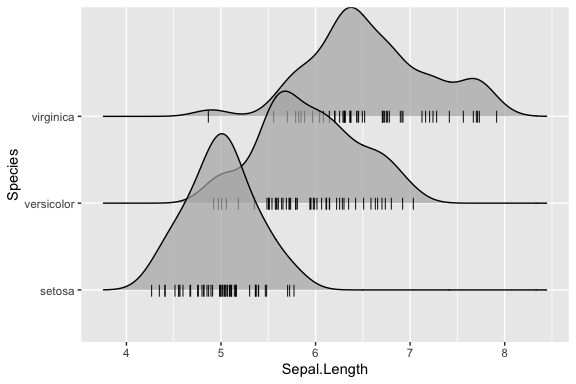Note that we are using `position_points_jitter()` here, not `position_jitter()`. We do this because `position_points_jitter()` knows to jitter only the points in a ridgeline plot, without touching the density lines.

Styling the jittered points is a bit tricky but is possible with special scales provided by ggridges. First, there is `scale_discrete_manual()` which can be used to make arbitrary discrete scales for arbitrary aesthetics. We use it in the next example to style the point shapes. Second, there are various point aesthetic scales, such as `scale_point_color_hue()`. See the reference documentation for these scales for more details.

``````ggplot(iris, aes(x = Sepal.Length, y = Species, fill = Species)) +
geom_density_ridges(
aes(point_color = Species, point_fill = Species, point_shape = Species),
alpha = .2, point_alpha = 1, jittered_points = TRUE
) +
scale_point_color_hue(l = 40) +
scale_discrete_manual(aesthetics = "point_shape", values = c(21, 22, 23))``````